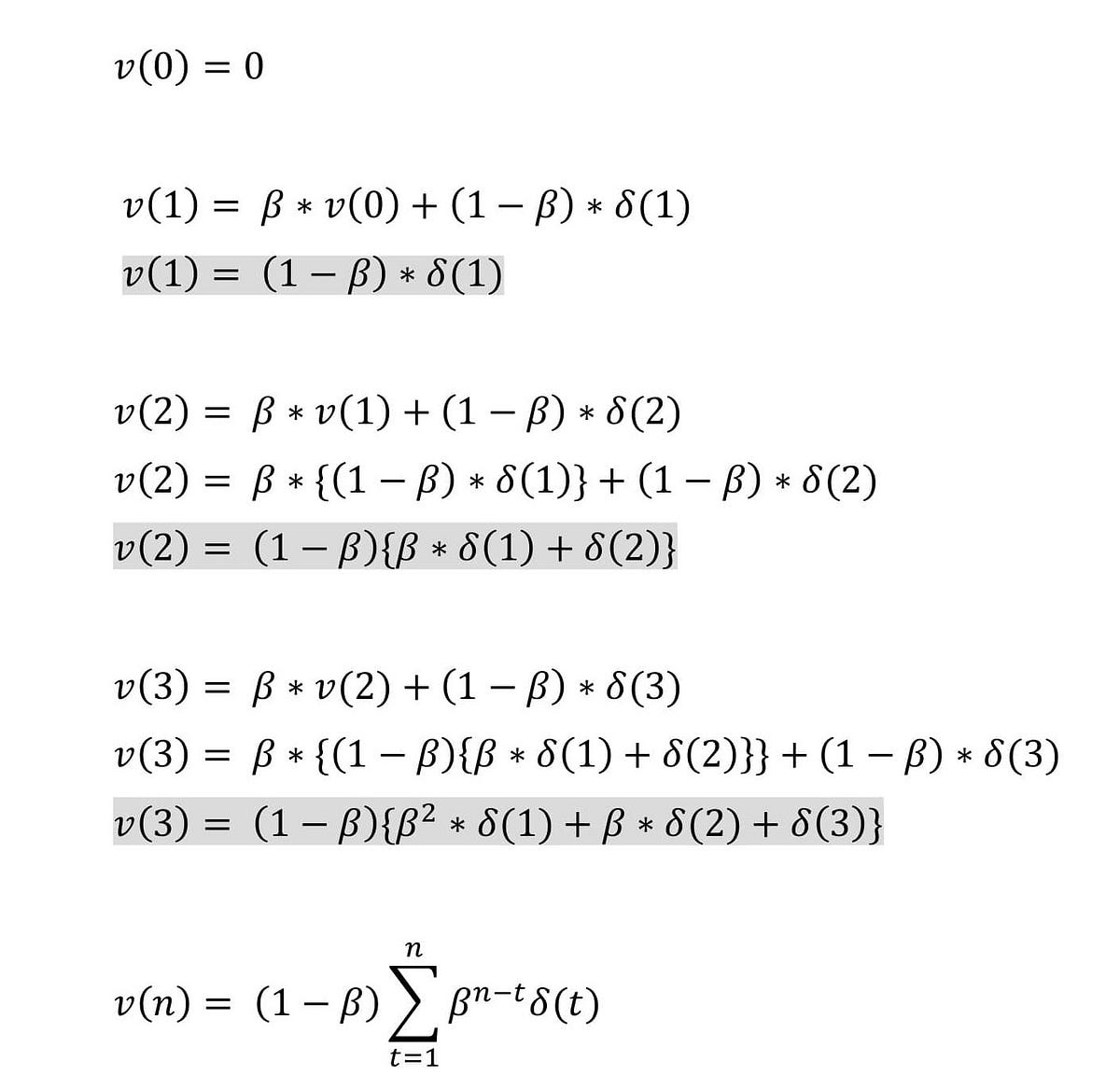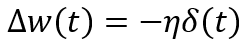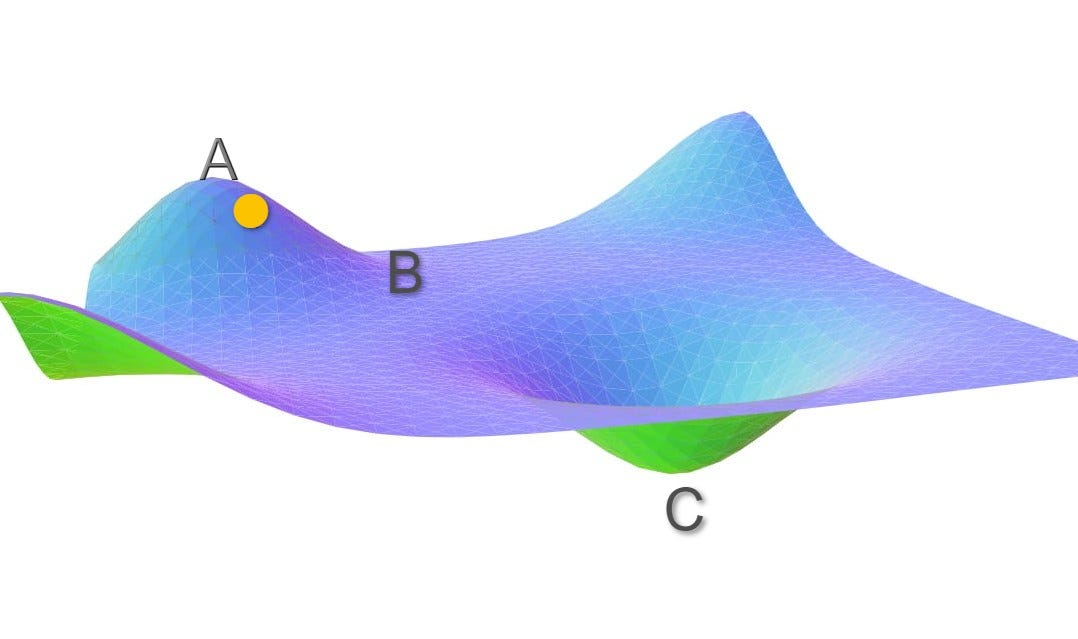The problem with vanilla gradient descent is that the weight update at a moment (t) is governed by the learning rate and gradient at that moment only. It doesn’t take into account the past steps taken while traversing the cost space.Image by author

It leads to the following problems.

1. The gradient of the cost function at saddle points( plateau) is negligible or zero, which in turn leads to small or no weight updates. Hence, the network becomes stagnant, and learning stops
2. The path followed by Gradient Descent around a steep valley is very jittery even when operating with mini-batch mode

Consider the below cost surface.Image by author

Let’s assume the initial weights of the network under consideration correspond to point A. With vanilla gradient descent, the Loss function decreases rapidly along the slope AB as the gradient along this slope is high. But as soon as it reaches point B the gradient becomes very low. The weight updates around B is very small. Even after many iterations, the cost moves very slowly before getting stuck at a point where the gradient eventually becomes zero.

In this case, ideally, cost should have moved to the global minima point C, but because the gradient disappears at point B, we are stuck with a sub-optimal solution.

## HTML entity encoder decoder Online

Ever wondered how the machine learning algorithms give us the optimal result, whether it is prediction, classification or any other? How…

## What is Supervised Machine Learning

What is neuron analysis of a machine? Learn machine learning by designing Robotics algorithm. Click here for best machine learning course models with AI

## Learning Rates and the Convergence of Gradient Descent

In the previous article we dived into the fundamental theory behind standard backpropagation and also introduced the different aspects that are responsible for practically optimizing the process.

## Pros and Cons of Machine Learning Language

AI, Machine learning, as its title defines, is involved as a process to make the machine operate a task automatically to know more join CETPA